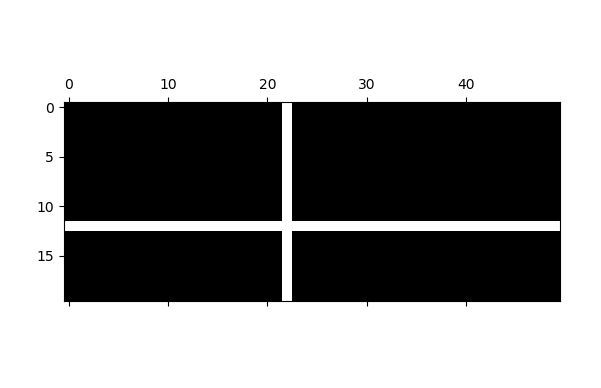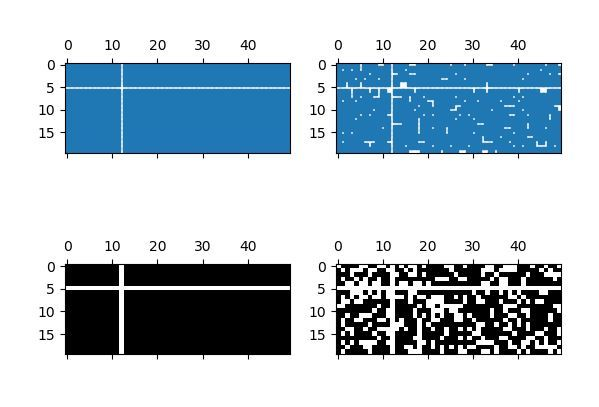Skip to content
Related Articles
Matplotlib.axes.Axes.spy() in Python
• Last Updated : 13 Apr, 2020

Matplotlib is a library in Python and it is numerical – mathematical extension for NumPy library. The Axes Class contains most of the figure elements: Axis, Tick, Line2D, Text, Polygon, etc., and sets the coordinate system. And the instances of Axes supports callbacks through a callbacks attribute.

## matplotlib.axes.Axes.spy() Function

The Axes.spy() function in axes module of matplotlib library is also used to plot the sparsity pattern of a 2D array.It is also used to visualize the non-zero values of the array.

Syntax: Axes.spy(self, Z, precision=0, marker=None, markersize=None, aspect=’equal’, origin=’upper’, **kwargs

Parameters: This method accept the following parameters that are described below:

• Z : This parameter is the array which is to be plotted.
• precision : This parameter is used to determine whether any non-zero value is to be plotted or not.
• origin : This parameter place the [0, 0] index of the array in the upper left or lower left corner of the axes.
• aspect : This parameter is an optional and it is used to control the aspect ratio of the axes.

Returns: This returns the following:

• ret:This returns the AxesImage or Line2D. And it depend on style of plotting

Below examples illustrate the matplotlib.axes.Axes.spy() function in matplotlib.axes:

Example-1:

 `# Implementation of matplotlib function``import` `matplotlib.pyplot as plt``import` `numpy as np`` ` `fig, ax1 ``=` `plt.subplots()`` ` `x ``=` `np.random.randn(``20``, ``50``)``x[``12``, :] ``=` `0.``x[:, ``22``] ``=` `0.``ax1.spy(x)`` ` `plt.show()``ax1.set_title(``'matplotlib.axes.Axes.spy() Example'``)``plt.show()`

Output:Example-2:

 `# Implementation of matplotlib function``import` `matplotlib.pyplot as plt``import` `numpy as np`` ` `fig, [(ax1, ax2), (ax3, ax4)] ``=` `plt.subplots(``2``, ``2``)`` ` `x ``=` `np.random.randn(``20``, ``50``)``x[``5``, :] ``=` `0.``x[:, ``12``] ``=` `0.`` ` `ax1.spy(x, markersize ``=` `4``)``ax2.spy(x, precision ``=` `0.2``, markersize ``=` `4``)`` ` `ax3.spy(x)``ax4.spy(x, precision ``=` `0.4``)`` ` `plt.show()``ax1.set_title(``'matplotlib.axes.Axes.spy() Example'``)``plt.show()`

Output:Attention geek! Strengthen your foundations with the Python Programming Foundation Course and learn the basics.

To begin with, your interview preparations Enhance your Data Structures concepts with the Python DS Course. And to begin with your Machine Learning Journey, join the Machine Learning – Basic Level Course

My Personal Notes arrow_drop_up